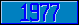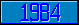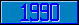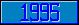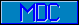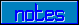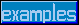## Relational operator 'follows' (])

Introduced in the 1977 ANSI M[UMPS] language standard.

Assume that TXT1="ABC" and TXT2="ABD".

Reference   Value
TXT2]TXT1   1 (true)
"ABCD"]TXT1   1 (true)

Note that the lexicographical order of the texts "1", "2" and "10" is: "1", "10", "2", i.e.:

Reference   Value
10]1   1 (true)
2]1   1 (true)
10]2   0 (false)